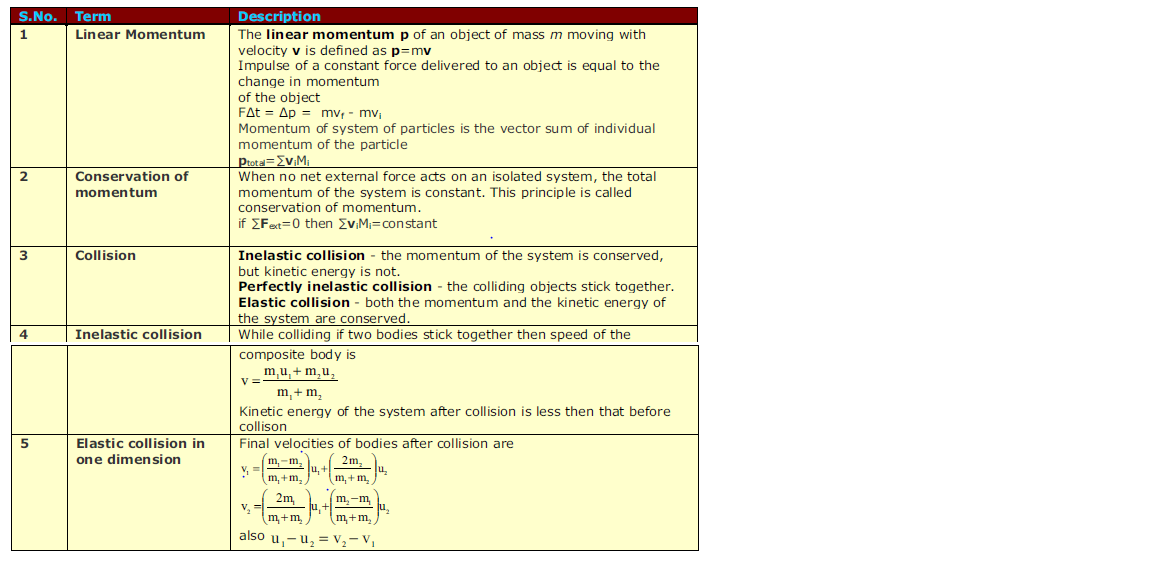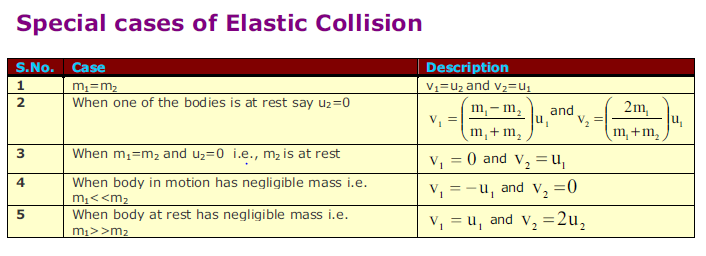Home » Competitive Exams » JEE advanced » How to effectively solve the Momentum and Collisions problems

# How to effectively solve the Momentum and Collisions problems

Things to remember for momentum problems
1) Law of conservation of Momentum. The total momentum for the isolated remains constant
2) Elastic collision: In this collison, Momentum and Kinetic energy of the system remains conserved
3) Inelastic collision: In this collision ,Momentum is conserved only

General approach to solve the momentum problems

• Choose your frame of reference
• Set up a coordinate system and define your velocities with respect to that system.It is usually convenient to have the x axis coincide with one of the initial velocities.
• In your sketch of the coordinate system, draw and label all velocity vectors and include all the given information.
• Write expressions for the x and y components of the momentum of each object before and after the collision. Remember to include the appropriate signs for the components of the velocity vectors.
• Write expressions for the total momentum in the x direction before and after the collision and equate the two. Repeat this procedure for the total momentum in the y direction. These steps follow from the fact that, because the momentum of the system is conserved in any collision(law on conservation of linear momentum), the total momentum along any direction must also be constant. Remember, it is the momentum of the system that is constant, not the momentan of the individual objects.
• If the collision is inelastic, kinetic energy is not conserved, and additional information is probably required. If the collision is perfectly inelastic, the final velocities of the two objects are equal. Solve the momentum equations for the unknown quantities.
• If the collision is elastic, kinetic energy is conserved, and you can equate the total kinetic energy before the collision to the total kinetic energy after the collision to get an additional relationship between the velocities.And you can solve the energy and momentum equation to find out the find velocities.
• Centre of mass can be useful feature in solving the momentum problems
• Remember we need to get as many equation as the number of unknown

Type of momentum problems
1) Two free-moving objects colliding
2) An object exploding into two or more pieces
3) Problems having elastic or inelastic collision

Some Quick Formula for standard momentum problems
a) One dimensional perfectly elastic collision

Law of conservation of momentum
Pf = Pi
Since the motion is One dimensional, Conservation of Energy becomes
v1f – v2f = –(v1i – v2i)

b) One dimensional totally inelastic collision
Law of conservation of momentum
Pf = Pi
Imp point vf is same for both the object

c) Two dimensional totally inelastic collision
Law of conservation of momentum in x and y axis
Pfx = Pix
Pfy = PiyDetailed Notes for Momentum and Collision can be found at

<iframe width=”560″ height=”315″ src=”https://www.youtube.com/embed/UERvKx-cqJs” frameborder=”0″ allowfullscreen></iframe>

<iframe width=”560″ height=”315″ src=”https://www.youtube.com/embed/HkaQb9nQCik” frameborder=”0″ allowfullscreen></iframe>